# Spectrum of spaces

(diff) ← Older revision | Latest revision (diff) | Newer revision → (diff)

A representing object for a generalized cohomology theory. The notion was introduced in  (cf. also Generalized cohomology theories).

A spectrum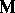of spaces is defined as a sequence of topological (as a rule cellular) spacestogether with mappings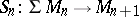, where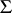denotes suspension. Spectra of spaces form a category; a morphism of a spectruminto a spectrum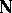is, roughly speaking, the "cofinal part" of some function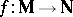given by a family of mappings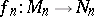with(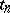is toasis to). The concepts of homotopic morphisms and homotopy equivalent spectra of spaces may be introduced, and one may construct the homotopy category of spectra . Postnikov systems (cf. Postnikov system) of spectra of spaces have also been introduced.

The suspension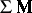over a spectrum of spacesis defined as the spectrum of spaces. Let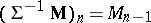. Thenand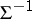are homotopically mutually inverse functors, so that in the category of spectra of spaces (in contrast to the category of spaces), the suspension functor is invertible, and this makes it convenient to work in the former. In general, in the category of spectra of spaces, all arguments connected with stability (e.g. the construction of the Adams spectral sequence) assume a natural form.

## Contents

### Examples of spectra of spaces.

1) For any spaceone can define a spectrum of spaces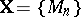, where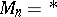when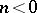and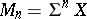when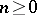, andis the natural identification. So, forone obtains the spectrum of spheres.

2) The spectrum of the Eilenberg–MacLane spaces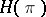(or), whereis an Abelian group. The homotopy equivalence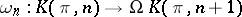, whereis an Eilenberg–MacLane space and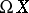is the loop space over, gives an adjoint mapping, so that one obtains the spectrum of spaces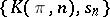. This spectrum of spaces represents the ordinary cohomology theory with coefficients in.

3) Letbe a space such thatfor some. For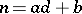,,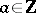, let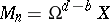. There arises a sequenceof the form. As in example 2), the homotopy equivalence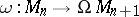gives a mapping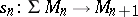, so that one obtains a spectrum of spaces. E.g., for the classifying space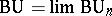, where theare unitary groups,(Bott's periodicity theorem), and one obtains the spectrum of spacesrepresenting complex-theory. An analogous result holds for real-theory (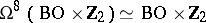).

4) Various Thom spectra (cf. Thom spectrum), representing cobordism theories.

Given two spectra of spacesand, one can define their reduced product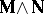(an analogue of the usual reduced product of spaces). A multiplication onis defined as a morphism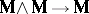which is associative (in the appropriate sense). A spectrum of spaces equipped with a multiplication is called a ring spectrum, or multiplicative spectrum, and the cohomology theory represented by it is multiplicative. Attempts to overcome the difficulties connected with the "poor associativity" of the above multiplication have led to a revision of the foundations of the theory of spectra of spaces. Namely, the concept of a coordinate-free spectrum of spaces is introduced as a family of spaces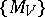(and corresponding mappings) indexed by linear subspacesof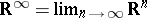. The category of coordinate-free spectra of spaces is isomorphic to the category of ordinary spectra of spaces, but the pairing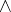in it is easier to control, and so it plays an important role in the examination of subtle geometric questions connected with the higher structures of spectra of spaces, with orientations in cohomology theory, and elsewhere.

How to Cite This Entry:
Spectrum of spaces. Encyclopedia of Mathematics. URL: http://encyclopediaofmath.org/index.php?title=Spectrum_of_spaces&oldid=12104
This article was adapted from an original article by Yu.B. Rudyak (originator), which appeared in Encyclopedia of Mathematics - ISBN 1402006098. See original article